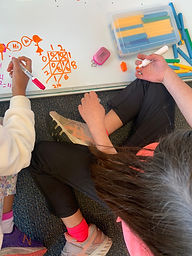Mr. Rodney

Target 1​

Lesson Type:

New

Number Operation

:

Computation

Multiply within 100.

1:

Understand that multiplication represents the combination of equal groups of objects.

2:

Interpret products of whole numbers as the total number of objects arranged into equal groupings.

3:

Understand that in multiplication equations, the first factor represents the number of groups and the second factor represents the quantity in each group.

4:

Understand that multiplying numbers makes numbers larger.

4th

Vocabulary:

Factor, Product, Lattice Multiplication

Activities:

Reviewed and discussed vocabulary terms.

Used base-10 blocks to visually represent and solve for a multiplication problem (multi-digit multiplied by a single digit).

By using the base-10 blocks, students gained a deeper understanding of why we regroup or "carry over" into the next place value.

For example, when multiplying 5 x 3 in the ones place, we have a product of 15--1 ten and 5 ones. We regroup the 1 into its corresponding place value.

Continued to practice multi-digit by single-digit multiplication, understanding that the same steps are used when multiplying twio-digit numbers by a single digit, or 5 digits by a single digit.

Explored "Lattice Multiplication" which allowed students to multiply multi-digit factors without having to worry about place holders as one would in the standard algorithm.Home Exploration

Challenge Problem:

Calculate the products for the given multiplication problems.

1) 221 x 4
2) 352 x 5
3) 473 x 6
4) 1254 x 3
5) 2972 x 8

Guiding Questions:

After writing our the problems, use dotted lines to help map out the place values.

• When regrouping a number into the next place value, it's a good practice to add a "+" in front of it as a reminder to add it to the product of that place value.
• If the problem requires you to regroup into a place value that is larger than any of the factors (as in problem 2, 3, and 5) your child may simply "drop" the number into the answer or go through the process of multiplying by 0 and then adding the carry-over. Either way is completely fine and acceptable!

1) 884
2) 1760
3) 2838
4) 3762
5) 23776Absent Students:

Target 2

:

Vocabulary:

Activities:Home Exploration

Guiding Questions:Target 3

:

Vocabulary:

Activities:Home Exploration